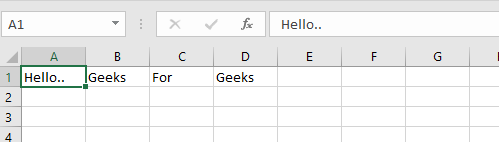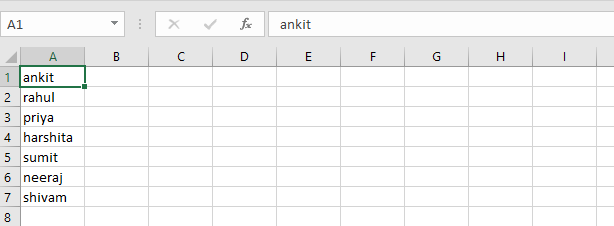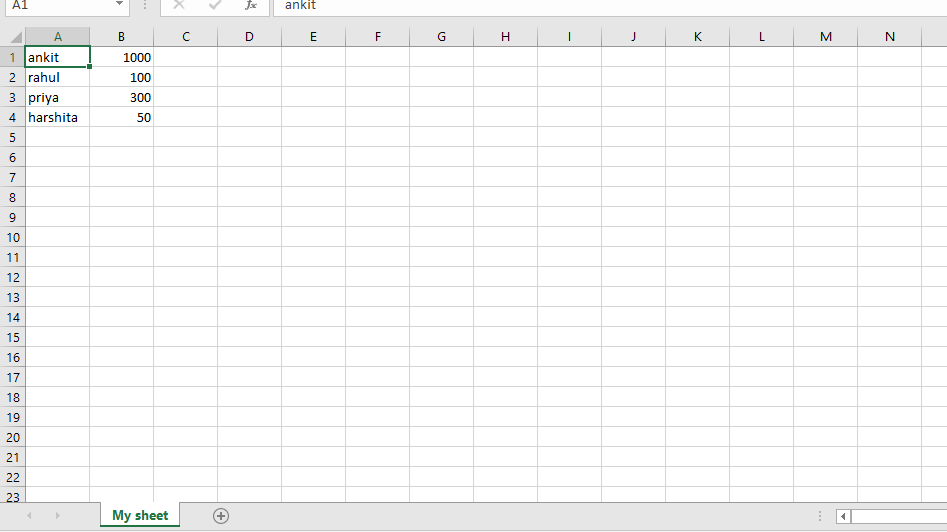Open In App

# Python | Create and write on excel file using xlsxwriter module

XlsxWriter is a Python module for writing files in the XLSX file format. It can be used to write text, numbers, and formulas to multiple worksheets. Also, it supports features such as formatting, images, charts, page setup, auto filters, conditional formatting and many others.
Use this command to install xlsxwriter module:

` pip install xlsxwriter `

Note: Throughout XlsxWriter, rows and columns are zero indexed. The first cell in a worksheet, A1 is (0, 0), B1 is (0, 1), A2 is (1, 0), B2 is (1, 1) ..similarly for all.
Let’s see how to create and write to an excel-sheet using Python.
Code #1 : Using A1 notation(cell name) for writing data in the specific cells.

## Python3

 `# import xlsxwriter module``import` `xlsxwriter` `# Workbook() takes one, non-optional, argument``# which is the filename that we want to create.``workbook ``=` `xlsxwriter.Workbook(``'hello.xlsx'``)` `# The workbook object is then used to add new``# worksheet via the add_worksheet() method.``worksheet ``=` `workbook.add_worksheet()` `# Use the worksheet object to write``# data via the write() method.``worksheet.write(``'A1'``, ``'Hello..'``)``worksheet.write(``'B1'``, ``'Geeks'``)``worksheet.write(``'C1'``, ``'For'``)``worksheet.write(``'D1'``, ``'Geeks'``)` `# Finally, close the Excel file``# via the close() method.``workbook.close()`

Output:Code #2 : Using the row-column notation(indexing value) for writing data in the specific cells.

## Python3

 `# import xlsxwriter module``import` `xlsxwriter` `workbook ``=` `xlsxwriter.Workbook(``'Example2.xlsx'``)``worksheet ``=` `workbook.add_worksheet()` `# Start from the first cell.``# Rows and columns are zero indexed.``row ``=` `0``column ``=` `0` `content ``=` `[``"ankit"``, ``"rahul"``, ``"priya"``, ``"harshita"``,``                    ``"sumit"``, ``"neeraj"``, ``"shivam"``]` `# iterating through content list``for` `item ``in` `content :` `    ``# write operation perform``    ``worksheet.write(row, column, item)` `    ``# incrementing the value of row by one``    ``# with each iterations.``    ``row ``+``=` `1``    ` `workbook.close()`

Output:Code #3 : Creating a new sheet with the specific name

## Python3

 `# import xlsxwriter module``import` `xlsxwriter` `workbook ``=` `xlsxwriter.Workbook(``'Example3.xlsx'``)` `# By default worksheet names in the spreadsheet will be``# Sheet1, Sheet2 etc., but we can also specify a name.``worksheet ``=` `workbook.add_worksheet(``"My sheet"``)` `# Some data we want to write to the worksheet.``scores ``=` `(``    ``[``'ankit'``, ``1000``],``    ``[``'rahul'``,   ``100``],``    ``[``'priya'``,  ``300``],``    ``[``'harshita'``,    ``50``],``)` `# Start from the first cell. Rows and``# columns are zero indexed.``row ``=` `0``col ``=` `0` `# Iterate over the data and write it out row by row.``for` `name, score ``in` `(scores):``    ``worksheet.write(row, col, name)``    ``worksheet.write(row, col ``+` `1``, score)``    ``row ``+``=` `1` `workbook.close()`

Output:XlsxWriter has some advantages and disadvantages over the alternative Python modules for writing Excel files.